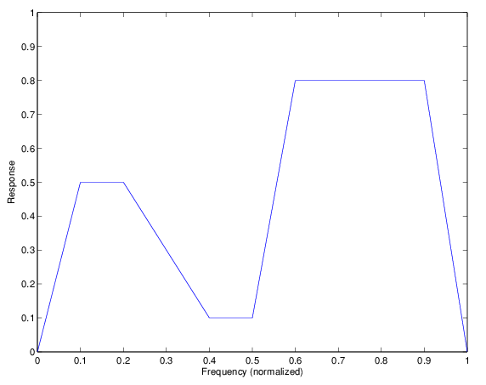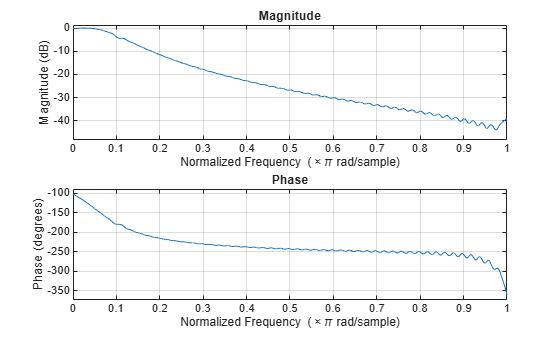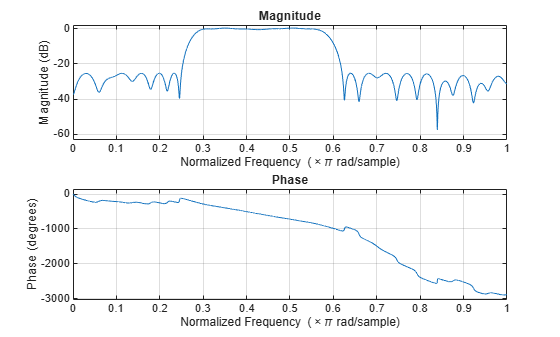Main Content

# fdesign.arbmagnphase

Arbitrary response magnitude and phase filter specification object

## Syntax

```d = fdesign.arbmagnphase d = fdesign.arbmagnphase(specification) d = fdesign.arbmagnphase(specification,specvalue1,specvalue2,...) d = fdesign.arbmagnphase(specvalue1,specvalue2,specvalue3) d = fdesign.arbmagnphase(...,fs) ```

## Description

`d = fdesign.arbmagnphase` constructs an arbitrary magnitude filter specification object `d`.

`d = fdesign.arbmagnphase(specification)` initializes the `Specification` property for specifications object `d` to `specification`. The input argument `specification` must be one of the choices shown in the following table. Specification options are not case sensitive.

Specification

Description of Resulting Filter

`n,f,h`

Single band design (default). FIR and IIR (`n` is the order for both numerator and denominator).

`n,b,f,h`

FIR multiband design where `b` defines the number of bands.

`nb,na,f,h`

IIR single band design.

The following table describes the specification arguments.

Argument

Description

`b`

Number of bands in the multiband filter.

`f`

Frequency vector. Frequency values specified in `f` indicate locations where you provide specific filter response amplitudes. When you provide `f` you must also provide `h` which contains the response values.

`h`

Complex frequency response values.

`n`

Filter order for FIR filters and the numerator and denominator orders for IIR filters (when not specified by `nb` and `na`).

`nb`

Numerator order for IIR filters.

`na`

Denominator order for IIR filter designs.

By default, this method assumes that all frequency specifications are supplied in normalized frequency.

### Specifying f and h

`f` and `h` are the input arguments you use to define the filter response desired. Each frequency value you specify in `f` must have a corresponding response value in `h`. This example creates a filter with two passbands (`b` = `4`) and shows how `f` and `h` are related. This example is for illustration only. It is not an actual filter.

Define the frequency vector `f` as ```[0 0.1 0.2 0.4 0.5 0.6 0.9 1.0]```

Define the response vector `h` as ```[0 0.5 0.5 0.1 0.1 0.8 0.8 0]```

These specifications connect`f` and `h` as shown in the following table.

f (Normalized Frequency)

h (Response Desired at f)

0

0

0.1

0.5

0.2

0.5

0.4

0.1

0.5

0.1

0.6

0.8

0.9

0.8

1.0

0.0

A response with two passbands—one roughly between 0.1 and 0.2 and the second between 0.6 and 0.9—results from the mapping between `f` and `h`. Plotting `f` and `h` yields the following figure that resembles a filter with two passbands.The second example in Examples shows this plot in more detail with a complex filter response for `h`. In the example, `h` uses complex values for the response.

Different specification types often have different design methods available. Use `designmethods``(d)` to get a list of design methods available for a given specification option and specifications object.

`d = fdesign.arbmagnphase(specification,specvalue1,specvalue2,...)` initializes the filter specification object with `specvalue1`, `specvalue2`, and so on. Use `get(d,'description')` for descriptions of the various specifications `specvalue1`, `specvalue2`, ...`spec``n`.

`d = fdesign.arbmagnphase(specvalue1,specvalue2,specvalue3)` uses the default specification option `n,f,h`, setting the filter order, filter frequency vector, and the complex frequency response vector to the values `specvalue1`, `specvalue2`, and `specvalue3`.

`d = fdesign.arbmagnphase(...,fs)` specifies the sampling frequency in Hz. All other frequency specifications are also assumed to be in Hz when you specify `fs`.

## Examples

collapse all

Use `fdesign.arbmagnphase` to model a complex analog filter.

```d = fdesign.arbmagnphase('n,f,h',100); % N=100, f and h set to defaults. design(d,'freqsamp','SystemObject',true);```For a more complex example, design a bandpass filter with low group delay by specifying the desired delay and using f and h to define the filter bands.

```n = 50; % Group delay of a linear phase filter would be 25. gd = 12; % Set the desired group delay for the filter. f1=linspace(0,.25,30); % Define the first stopband frequencies. f2=linspace(.3,.56,40);% Define the passband frequencies. f3=linspace(.62,1,30); % Define the second stopband frequencies. h1 = zeros(size(f1)); % Specify the filter response at the freqs in f1. h2 = exp(-1j*pi*gd*f2); % Specify the filter response at the freqs in f2. h3 = zeros(size(f3)); % Specify the response at the freqs in f3. d=fdesign.arbmagnphase('n,b,f,h',50,3,f1,h1,f2,h2,f3,h3); D = design(d,'equiripple','SystemObject',true); fvtool(D,'Analysis','freq');```## See Also

Introduced in R2011a

## Support